Question

3-5

Oslo Company prepared the following contribution format income statement based on a sales volume of 1,000 units (the relevant range of production is 500 units to 1,500 units):

 Sales \$ 80,000 Variable expenses 52,000 Contribution margin 28,000 Fixed expenses 21,840 Net operating income \$ 6,160

If sales decline to 900 units, what would be the net operating income?

Sales price per unit=80,000/1000=\$80 per unit

Variable cost per unit=52,000/1000=\$52 per unit

 Sales(80*900) 72,000 Variable expenses(52*900) 46800 Contribution margin 25200 Fixed expenses 21840 Net operating income \$3360.

NOTE:Total fixed costs and variable cost per unit do not change with change in units

#### Earn Coins

Coins can be redeemed for fabulous gifts.

Similar Homework Help Questions
• ### Oslo Company prepared the following contribution format income statement based on a sales volume of 1,000...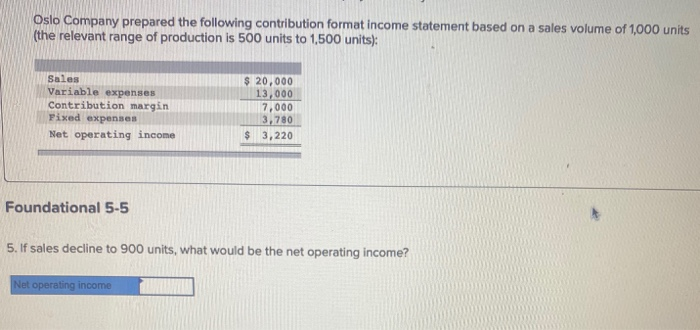Oslo Company prepared the following contribution format income statement based on a sales volume of 1,000 units (the relevant range of production is 500 units to 1,500 units): Sales Variable expenses Contribution margin Fixed expenses Net operating income \$ 20,000 13,000 7,000 3,780 \$ 3,220 Foundational 5-5 5. If sales decline to 900 units, what would be the net operating income? Net operating income Oslo Company prepared the following contribution format income statement based on a sales volume of 1,000...

• ### Oslo Company prepared the following contribution format income statement based on a sales volume of 1,000...

Oslo Company prepared the following contribution format income statement based on a sales volume of 1,000 units (the relevant range of production is 500 units to 1,500 units): Sales \$ 55,000 Variable expenses 33,000 Contribution margin 22,000 Fixed expenses 14,960 Net operating income \$ 7,040 5. If sales decline to 900 units, what would be the net operating income?

• ### Required Information The following information applies to the questions displayed below.] Oslo Company prepared the following...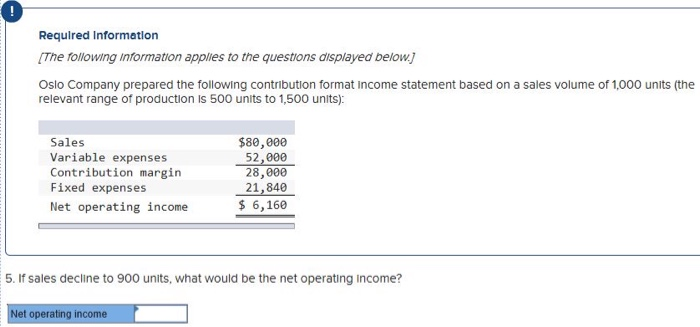Required Information The following information applies to the questions displayed below.] Oslo Company prepared the following contribution format relevant range of production is 500 units to 1,500 units): Sales Variable expenses Contribution margin Fixed expenses Net operating income \$80,000 52,000 28,000 21,840 \$ 6,160 5. If sales decline to 900 units, what would be the net operating income? Net operating income

• ### Required Information The following information applies to the questions displayed below. Oslo Company prepared the following...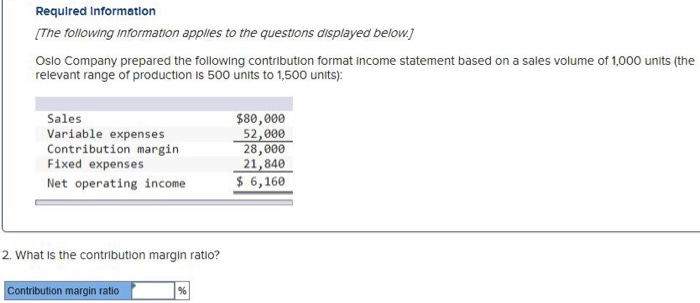Required Information The following information applies to the questions displayed below. Oslo Company prepared the following contribution format Income statement based on a sales volume of 1,000 units (the relevant range of production is 500 units to 1,500 units): Sales Variable expenses Contribution margin Fixed expenses Net operating income \$80,000 52,000 28,000 21,840 \$ 6,160 2. What is the contribution margin ratio? Contribution margin ratio

• ### Requlred Information The following information applies to the questions displayed below) Oslo Company prepared the following...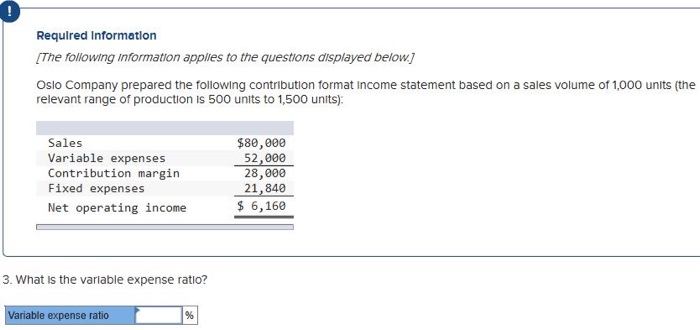Requlred Information The following information applies to the questions displayed below) Oslo Company prepared the following contribution format Income statement based on a sales volume of 1,000 units (the relevant range of production is 500 units to 1,500 units): Sales Variable expenses Contribution margin Fixed expenses Net operating income \$80,000 52,000 28,000 21,840 \$ 6,160 3. What is the variable expense ratio? Variable expense ratio %

• ### Required information The following information applies to the questions displayed below.) Oslo Company prepared the following...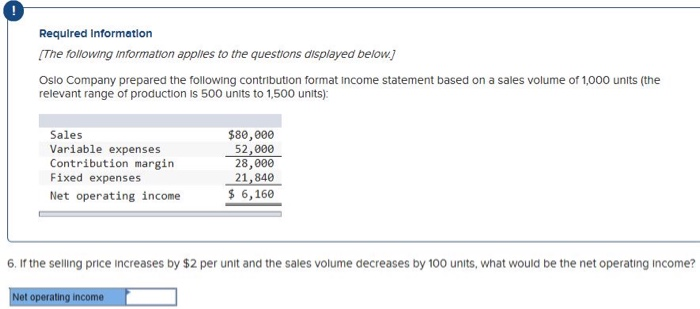Required information The following information applies to the questions displayed below.) Oslo Company prepared the following contribution format income statement based on a sales volume of 1,000 units (the relevant range of production is 500 units to 1,500 units): Sales Variable expenses Contribution margin Fixed expenses Net operating income \$80,000 52,000 28,000 21,840 \$ 6,160 6. If the selling price increases by \$2 per unit and the sales volume decreases by 100 units, what would be the net operating income?...

• ### Required Information [The following information applies to the questions displayed below) Oslo Company prepared the following...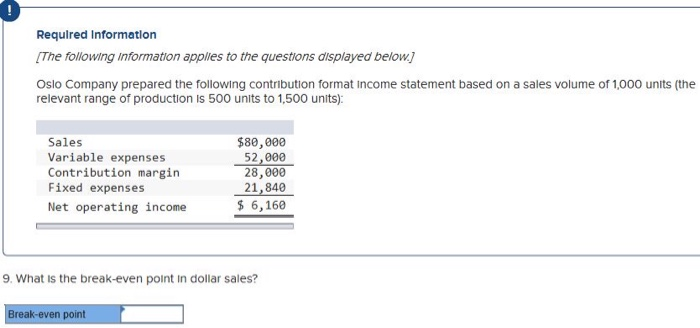Required Information [The following information applies to the questions displayed below) Oslo Company prepared the following contribution format Income statement based on a sales volume of 1,000 units (the relevant range of production is 500 units to 1,500 units): Sales Variable expenses Contribution margin Fixed expenses Net operating income \$80,000 52,000 28,000 21,840 \$ 6,160 9. What is the break-even point in dollar sales? Break-even point

• ### Required Information The following information applies to the questions displayed below. Oslo Company prepared the following...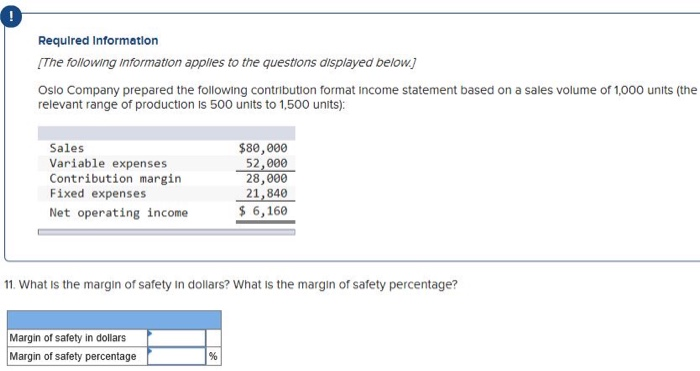Required Information The following information applies to the questions displayed below. Oslo Company prepared the following contribution format income statement based on a sales volume of 1,000 units (the relevant range of production is 500 units to 1,500 units): Sales Variable expenses Contribution margin Fixed expenses Net operating income \$80,000 52,000 28,000 21,840 \$ 6,160 11. What is the margin of safety in dollars? What is the margin of safety percentage? Margin of safety in dollars Margin of safety percentage

• ### Required Information The following Information applies to the questions displayed below.) Oslo Company prepared the following...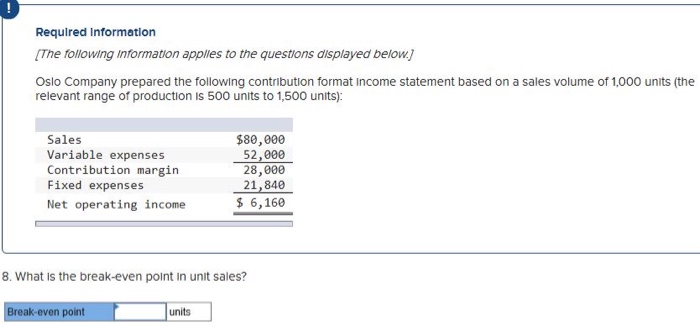Required Information The following Information applies to the questions displayed below.) Oslo Company prepared the following contribution format Income statement based on a sales volume of 1,000 units (the relevant range of production is 500 units to 1,500 units): Sales Variable expenses Contribution margin Fixed expenses Net operating income \$80,000 52,000 28,000 21,840 \$ 6,160 8. What is the break-even point in unit sales? Break-even point units units

• ### Oslo Company prepared the following contribution format income statement based on a sales volume of 1,000...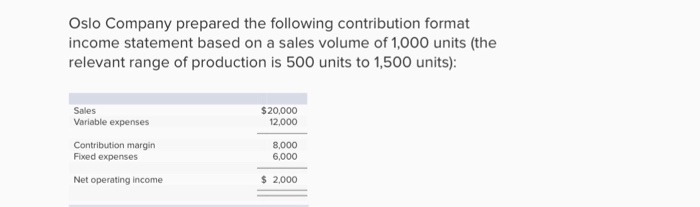Oslo Company prepared the following contribution format income statement based on a sales volume of 1,000 units (the relevant range of production is 500 units to 1,500 units) Sales Variable expenses \$20,000 12,000 Contribution margin Fixed expenses 8,000 6,000 Net operating income \$ 2,000